# Nuclear Physics MCQ Level – 2

## 10 Questions MCQ Test Modern Physics for IIT JAM | Nuclear Physics MCQ Level – 2

Description
This mock test of Nuclear Physics MCQ Level – 2 for Physics helps you for every Physics entrance exam. This contains 10 Multiple Choice Questions for Physics Nuclear Physics MCQ Level – 2 (mcq) to study with solutions a complete question bank. The solved questions answers in this Nuclear Physics MCQ Level – 2 quiz give you a good mix of easy questions and tough questions. Physics students definitely take this Nuclear Physics MCQ Level – 2 exercise for a better result in the exam. You can find other Nuclear Physics MCQ Level – 2 extra questions, long questions & short questions for Physics on EduRev as well by searching above.
QUESTION: 1

### Suppose that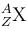decays by natural radioactivity in two stages to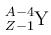the two stages would most likely be

Solution: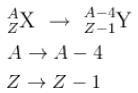Mass number reduces by 4 and number of protons by 1
option 1 says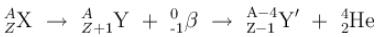∴  The first stage would be a β- decay and the second would be α emission.

The correct answer is:  β- emission with an anti neutrino  → α  emission

QUESTION: 2

Solution:

The correct answer is: random process

QUESTION: 3

### Why do heavier nuclei have a greater ratio of neutrons to protons than lighter nuclei?

Solution:

The correct answer is: to provide more attractive strong nuclear force to balance the repulsive electromagnetic force.

QUESTION: 4

Which of the following nuclei has the largest Binding Energy/nucleon?

(Consider the most abundant isotope of each)

Solution:

QUESTION: 5

The 238U nucleus has a binding energy of about 7.6 MeV/nucleon. If the nucleus were to fission into 2 equal fragments, each would have a K.E. of just over 100 MeV. From this, it can be concluded that

Solution:

The binding energy is the energy that keeps a nucleus together and makes its mass slightly different than its constituent particles.

If its masses were exactly the same as its constituent particles, its B.E would be 0 and it would be unstable.

Now, since the nucleus fissions into 2 equal fragmens.

∴  Each would have a mass number half of that of parent nucleus.

i.e.  A = 119 for each daughter nucleus. The difference in the Binding energies of the product and parent nucleus would be the K.E of the products.

If we assume that the daughter nucleus (each with A = 119 or A ~ 120) has a B.E of x per nucleon.

∴    –7.6 × 238 + x × 119 + x × 119 = 200 MeV
(–7.6 + x) 238 = 200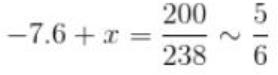–7.6 + x = 0.83
x = 7.6 + 0.83
x = 8.43
~ 8.5 MeV/nucleus

The correct answer is: Nuclei near A = 120 must be bound by about 8.5 MeV/nucleon.

QUESTION: 6

When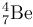transforms into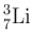does so by

Solution:

Use the process of elimination.

An  α  decay would change the atomic number from 7. So it cannot be option 1. A beta decay requires 2 particles to be emitted e / e+ and r / r option 2 and 3 don’t satisfy this. This leaves us with the 4th option.

The correct answer is: Electron capture by the nucleus with emission of a neutrino.

QUESTION: 7

Consider a heavy nucleus with spin 1/2 the magnitude of the ratio of the intrinsic magnetic moment of this nucleus to that of an electron is

Solution:

Since the Nucleus and e  have the same spin.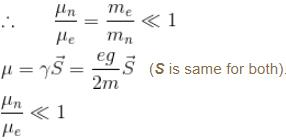Because mass of nucleus >> mass of e

The correct answer is: less than 1, because the nucleus has a mass much larger than that of the electron

QUESTION: 8

A Uranium nucleus decays at rest into a Thorium nucleus, as shown.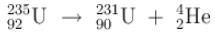Which of the following is true?

Solution:

Since the U nucleus decays at rest.

∴  The products have equal and opposite value of momenta.

The product with greater mass will have lower K.E.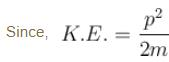The correct answer is: The Helium nucleus has more kinetic energy than the Thorium nucleus.

QUESTION: 9

The primary source of sun’s energy is a series of thermo nuclear reactions in which the energy produced is c2  times the mass difference between

Solution:

The reactions leading to production of energy in the sun are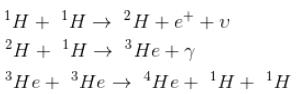i.e. 4 Hydrogen atoms and 1 Helium atom.
The correct answer is: 4 Hydrogen atoms and 1 Helium atom.

QUESTION: 10

Internal conversion is a process where by an excited nucleus transfers its energy directly to one of the most tightly bound atomic electrons, causing the electron to be ejected from the atom and leaving the atom in an excited state. The most probable process after an internal conversion electron is ejected from an atom with a high atomic number is that the

Solution:

Since the internal conversion leaves the atom in the excited state (and not the nucleus).

∴  option 2 seems most probable

The correct answer is: atom emits one or several X-rays